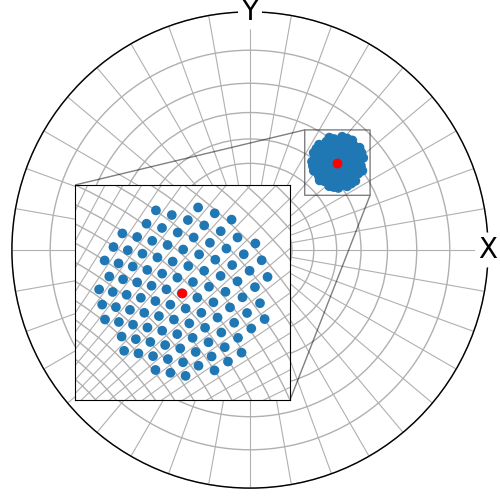# Zoom region inset#

This example shows how to add a region in the stereographic projection as a zoomed inset inside another stereographic projection, following the procedure in this Matplotlib example.```import matplotlib.pyplot as plt
from mpl_toolkits.axes_grid1.inset_locator import InsetPosition

from orix import plot, projections, sampling
from orix.vector import Vector3d

# Sample some orientations
v = sampling.sample_S2(2)
v_ref = Vector3d([1, 1, 1])
v2 = v[v_ref.angle_with(v, degrees=True) < 10]

# Plot them in the stereographic projection with a grid resolution of 10
# degrees
fig = plt.figure(figsize=(5, 5))
ax.stereographic_grid(True)
ax.set_labels("X", "Y", None, fontsize=20)
ax.scatter(v2)
ax.scatter(v_ref, c="r")

# Define some vectors describing the x/y extent of the zoomed inset
# region and get their stereographic coordinates (X, Y)
v_inset = Vector3d.from_polar(azimuth=[45, 45], polar=[36, 71], degrees=True)
stereo = projections.StereographicProjection()
x_inset, y_inset = stereo.vector2xy(v_inset)

# The zoomed inset rectangle origin (x, y), width and height
rect = [0.15, 0.2, 0.43, 0.43]

# Add a new stereographic projection axis with a grid resolution of 2
# degrees to the figure and plot the vectors here as well
ax_inset.stereographic_grid(True, 2, 2)
ax_inset.scatter(v2)
ax_inset.scatter(v_ref, c="r")

# Restrict the inset region to the extent vectors
ax_inset.set_xlim(x_inset.min(), x_inset.max())
ax_inset.set_ylim(y_inset.min(), y_inset.max())

# Add lines indicating the inset zoom
ip = InsetPosition(ax, rect)
ax_inset.set_axes_locator(ip)
ax.indicate_inset_zoom(ax_inset, edgecolor="k")

# Add border to the inset region
for spine in ax_inset.spines.values():
spine.set_visible(True)

# The inset axis is not compatible with fig.tight_layout(), so we set
# the origin and width and height manually